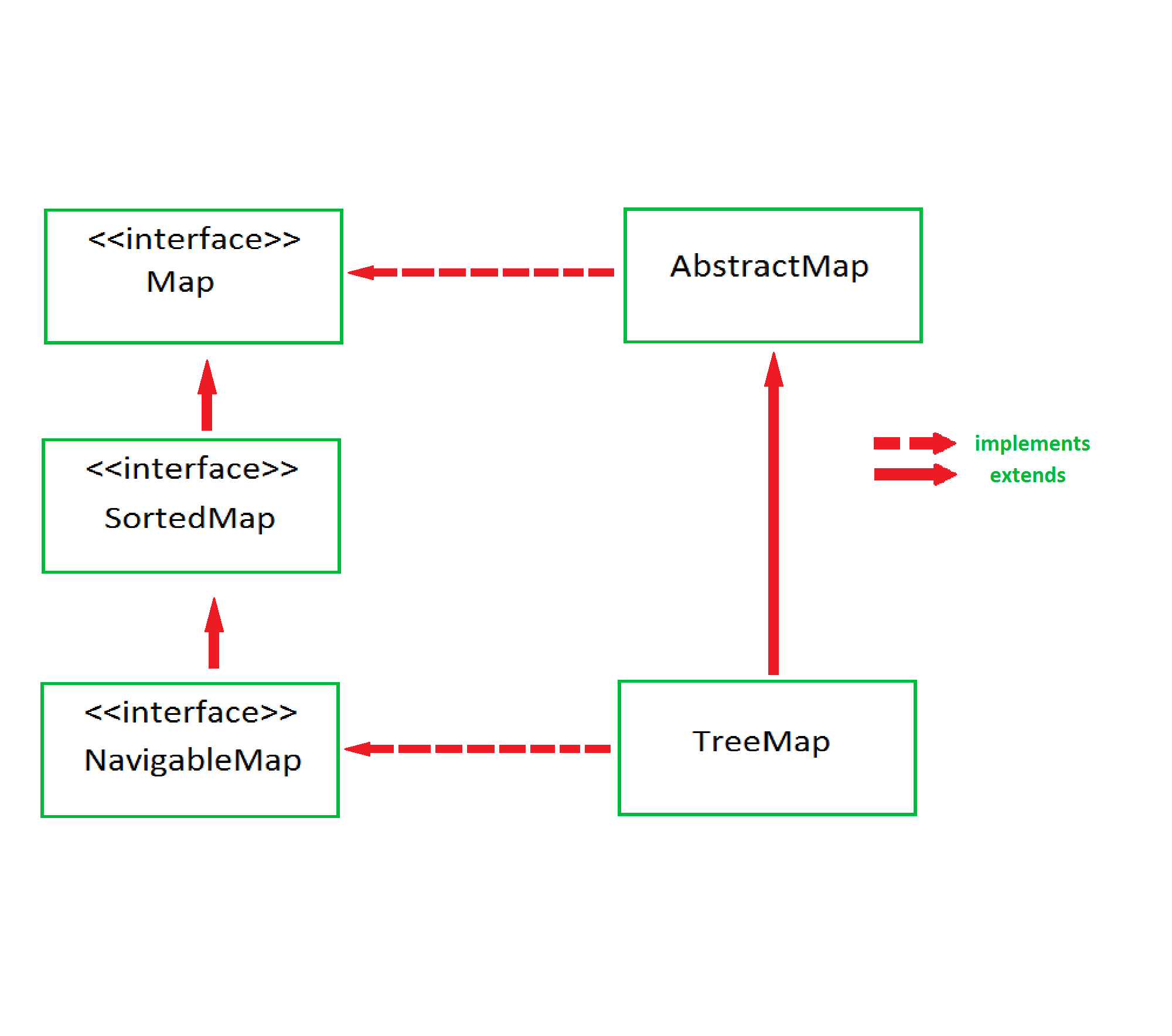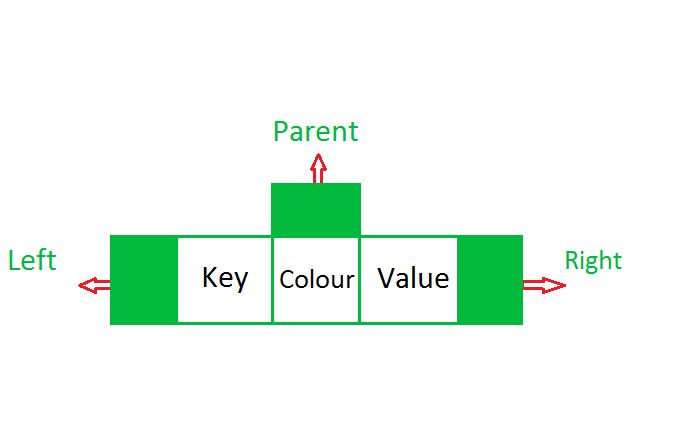# TreeMap in Java

The TreeMap in Java is used to implement Map interface and NavigableMap along with the Abstract Class. The map is sorted according to the natural ordering of its keys, or by a Comparator provided at map creation time, depending on which constructor is used. This proves to be an efficient way of sorting and storing the key-value pairs. The storing order maintained by the treemap must be consistent with equals just like any other sorted map, irrespective of the explicit comparators. The treemap implementation is not synchronized in the sense that if a map is accessed by multiple threads, concurrently and at least one of the threads modifies the map structurally, it must be synchronized externally. Some important features of the treemap are:

• This class is a member of Java Collections Framework.
• The class implements Map interfaces including NavigableMap, SortedMap and extends AbstractMap
• TreeMap in Java does not allow null keys (like Map) and thus a NullPointerException is thrown. However, multiple null values can be associated with different keys.
• All Map.Entry pairs returned by methods in this class and its views represent snapshots of mappings at the time they were produced. They do not support the Entry.setValue method.Performance factors:

`SortedMap m = Collections.synchronizedSortedMap(new TreeMap(...));`

Internal structure: The methods in TreeMap while getting keyset and values, return Iterator that are fail-fast in nature, thus any concurrent modification will throw ConcurrentModificationException.

TreeMap is based upon tree data structure. Each node in the tree has,

• 3 Variables (K key=Key, V value=Value, boolean color=Color)
• 3 References (Entry left = Left, Entry right = Right, Entry parent = Parent)Constructors in TreeMap:

1. TreeMap() : Constructs an empty tree map that will be sorted by using the natural order of its keys.

Example:

 `// Java code to show example of TreeMap ` ` `  `import` `java.util.*; ` `import` `java.util.concurrent.*; ` ` `  `public` `class` `TreeMapImplementation { ` ` `  `    ``// Function to show TreeMap() constructor example ` `    ``static` `void` `Example1stConstructor() ` `    ``{ ` `        ``// Creating an empty TreeMap ` `        ``TreeMap tree_map ` `            ``= ``new` `TreeMap(); ` ` `  `        ``// Mapping string values to int keys ` `        ``tree_map.put(``10``, ``"Geeks"``); ` `        ``tree_map.put(``15``, ``"4"``); ` `        ``tree_map.put(``20``, ``"Geeks"``); ` `        ``tree_map.put(``25``, ``"Welcomes"``); ` `        ``tree_map.put(``30``, ``"You"``); ` ` `  `        ``// Displaying the TreeMap ` `        ``System.out.println(``"TreeMap: "` `                           ``+ tree_map); ` `    ``} ` ` `  `    ``// Driver code ` `    ``public` `static` `void` `main(String[] args) ` `    ``{ ` ` `  `        ``System.out.println(``"TreeMap using "` `                           ``+ ``"TreeMap() constructor:\n"``); ` `        ``Example1stConstructor(); ` `    ``} ` `} `

Output:

```TreeMap using TreeMap() constructor:

TreeMap: {10=Geeks, 15=4, 20=Geeks, 25=Welcomes, 30=You}
```
2. TreeMap(Comparator comp) : Constructs an empty tree-based map that will be sorted by using the Comparator comp.

Example:

 `// Java code to show example of TreeMap ` ` `  `import` `java.util.*; ` `import` `java.util.concurrent.*; ` ` `  `// A class to represent a student. ` `class` `Student { ` `    ``int` `rollno; ` `    ``String name, address; ` ` `  `    ``// Constructor ` `    ``public` `Student(``int` `rollno, String name, ` `                   ``String address) ` `    ``{ ` `        ``this``.rollno = rollno; ` `        ``this``.name = name; ` `        ``this``.address = address; ` `    ``} ` ` `  `    ``// Used to print student details in main() ` `    ``public` `String toString() ` `    ``{ ` `        ``return` `this``.rollno + ``" "` `            ``+ ``this``.name + ``" "` `            ``+ ``this``.address; ` `    ``} ` `} ` ` `  `// Comparator implementattion ` `class` `Sortbyroll ``implements` `Comparator { ` ` `  `    ``// Used for sorting in ascending order of ` `    ``// roll number ` `    ``public` `int` `compare(Student a, Student b) ` `    ``{ ` `        ``return` `a.rollno - b.rollno; ` `    ``} ` `} ` ` `  `public` `class` `TreeMapImplementation { ` ` `  `    ``static` `void` `Example2ndConstructor() ` `    ``{ ` `        ``// Creating an empty TreeMap ` `        ``TreeMap tree_map ` `            ``= ``new` `TreeMap(``new` `Sortbyroll()); ` ` `  `        ``// Mapping string values to int keys ` `        ``tree_map.put(``new` `Student(``111``, ``"bbbb"``, ``"london"``), ``2``); ` `        ``tree_map.put(``new` `Student(``131``, ``"aaaa"``, ``"nyc"``), ``3``); ` `        ``tree_map.put(``new` `Student(``121``, ``"cccc"``, ``"jaipur"``), ``1``); ` ` `  `        ``// Displaying the TreeMap ` `        ``System.out.println(``"TreeMap: "` `                           ``+ tree_map); ` `    ``} ` ` `  `    ``public` `static` `void` `main(String[] args) ` `    ``{ ` ` `  `        ``System.out.println(``"TreeMap using "` `                           ``+ ``"TreeMap(Comparator)"` `                           ``+ ``" constructor:\n"``); ` `        ``Example2ndConstructor(); ` `    ``} ` `} `

Output:

```TreeMap using TreeMap(Comparator) constructor:

TreeMap: {111 bbbb london=2, 121 cccc jaipur=1, 131 aaaa nyc=3}
```
3. TreeMap(Map m) : Initializes a tree map with the entries from m, which will be sorted by using the natural order of the keys.

Example:

 `// Java code to show example of TreeMap ` ` `  `import` `java.util.*; ` `import` `java.util.concurrent.*; ` ` `  `public` `class` `TreeMapImplementation { ` ` `  `    ``static` `void` `Example3rdConstructor() ` `    ``{ ` `        ``// Creating a Map ` `        ``Map hash_map ` `            ``= ``new` `HashMap(); ` ` `  `        ``// Mapping string values to int keys ` `        ``hash_map.put(``10``, ``"Geeks"``); ` `        ``hash_map.put(``15``, ``"4"``); ` `        ``hash_map.put(``20``, ``"Geeks"``); ` `        ``hash_map.put(``25``, ``"Welcomes"``); ` `        ``hash_map.put(``30``, ``"You"``); ` ` `  `        ``// Creating the TreeMap using the Map ` `        ``TreeMap tree_map ` `            ``= ``new` `TreeMap(hash_map); ` ` `  `        ``// Displaying the TreeMap ` `        ``System.out.println(``"TreeMap: "` `                           ``+ tree_map); ` `    ``} ` ` `  `    ``public` `static` `void` `main(String[] args) ` `    ``{ ` ` `  `        ``System.out.println(``"TreeMap using "` `                           ``+ ``"TreeMap(Map)"` `                           ``+ ``" constructor:\n"``); ` `        ``Example3rdConstructor(); ` `    ``} ` `} `

Output:

```TreeMap using TreeMap(Map) constructor:

TreeMap: {10=Geeks, 15=4, 20=Geeks, 25=Welcomes, 30=You}
```
4. TreeMap(SortedMap sm) : Initializes a tree map with the entries from sm, which will be sorted in the same order as sm.

Example:

 `// Java code to show example of TreeMap ` ` `  `import` `java.util.*; ` `import` `java.util.concurrent.*; ` ` `  `public` `class` `TreeMapImplementation { ` ` `  `    ``// Function to show ` `    ``// TreeMap(SortedMap) constructor example ` `    ``static` `void` `Example4thConstructor() ` `    ``{ ` `        ``// Creating a SortedMap ` `        ``SortedMap sorted_map ` `            ``= ``new` `ConcurrentSkipListMap(); ` ` `  `        ``// Mapping string values to int keys ` `        ``sorted_map.put(``10``, ``"Geeks"``); ` `        ``sorted_map.put(``15``, ``"4"``); ` `        ``sorted_map.put(``20``, ``"Geeks"``); ` `        ``sorted_map.put(``25``, ``"Welcomes"``); ` `        ``sorted_map.put(``30``, ``"You"``); ` ` `  `        ``// Creating the TreeMap using the SortedMap ` `        ``TreeMap tree_map ` `            ``= ``new` `TreeMap(sorted_map); ` ` `  `        ``// Displaying the TreeMap ` `        ``System.out.println(``"TreeMap: "` `                           ``+ tree_map); ` `    ``} ` ` `  `    ``// Driver code ` `    ``public` `static` `void` `main(String[] args) ` `    ``{ ` ` `  `        ``System.out.println(``"TreeMap using "` `                           ``+ ``"TreeMap(SortedMap)"` `                           ``+ ``" constructor:\n"``); ` `        ``Example4thConstructor(); ` `    ``} ` `} `

Output:

```TreeMap using TreeMap(SortedMap) constructor:

TreeMap: {10=Geeks, 15=4, 20=Geeks, 25=Welcomes, 30=You}
```

Time Complexity: The algorithmic implementation is adapted from those of Red-Black Tree in Introduction to Algorithms (Eastern Economy Edition)This provides guaranteedtime cost for the containsKey, get, put and remove operations.

Methods of TreeMap:

1. boolean containsKey(Object key): Returns true if this map contains a mapping for the specified key.
2. boolean containsValue(Object value): Returns true if this map maps one or more keys to the specified value.
3. Object firstKey(): Returns the first (lowest) key currently in this sorted map.
4. Object get(Object key): Returns the value to which this map maps the specified key.
5. Object lastKey(): Returns the last (highest) key currently in this sorted map.
6. Object remove(Object key): Removes the mapping for this key from this TreeMap if present.
7. void putAll(Map map): Copies all of the mappings from the specified map to this map.
8. Set entrySet(): Returns a set view of the mappings contained in this map.
9. int size(): Returns the number of key-value mappings in this map.
10. Collection values(): Returns a collection view of the values contained in this map.
11. Object clone(): The method returns a shallow copy of this TreeMap.
12. void clear(): The method removes all mappings from this TreeMap and clears the map.
13. SortedMap headMap(Object key_value): The method returns a view of the portion of the map strictly less than the parameter key_value.
14. Set keySet(): The method returns a Set view of the keys contained in the treemap.
15. Object put(Object key, Object value): The method is used to insert a mapping into a map
16. SortedMap subMap((K startKey, K endKey): The method returns the portion of this map whose keys range from startKey, inclusive, to endKey, exclusive.
17. Object firstKey(): The method returns the first key currently in this tree map.

Example to insert, search and traversal in TreeMap:

 `// Java code to show creation, insertion, ` `// searching, and traversal in a TreeMap ` ` `  `import` `java.util.*; ` `import` `java.util.concurrent.*; ` ` `  `public` `class` `TreeMapImplementation { ` ` `  `    ``// Declaring a TreeMap ` `    ``static` `TreeMap tree_map; ` ` `  `    ``// Function to create TreeMap ` `    ``static` `void` `create() ` `    ``{ ` `        ``// Creating an empty TreeMap ` `        ``tree_map ` `            ``= ``new` `TreeMap(); ` ` `  `        ``System.out.println(``"TreeMap successfully"` `                           ``+ ``" created"``); ` `    ``} ` ` `  `    ``// Function to Insert values in the TreeMap ` `    ``static` `void` `insert() ` `    ``{ ` `        ``// Mapping string values to int keys ` `        ``tree_map.put(``10``, ``"Geeks"``); ` `        ``tree_map.put(``15``, ``"4"``); ` `        ``tree_map.put(``20``, ``"Geeks"``); ` `        ``tree_map.put(``25``, ``"Welcomes"``); ` `        ``tree_map.put(``30``, ``"You"``); ` ` `  `        ``System.out.println(``"\nElements successfully"` `                           ``+ ``" inserted in the TreeMap"``); ` `    ``} ` ` `  `    ``// Function to search a key in TreeMap ` `    ``static` `void` `search(``int` `key) ` `    ``{ ` ` `  `        ``// Checking for the key ` `        ``System.out.println(``"\nIs key \""` `+ key + ``"\" present? "` `                           ``+ tree_map.containsKey(key)); ` `    ``} ` ` `  `    ``// Function to search a value in TreeMap ` `    ``static` `void` `search(String value) ` `    ``{ ` ` `  `        ``// Checking for the value ` `        ``System.out.println(``"\nIs value \""` `+ value + ``"\" present? "` `                           ``+ tree_map.containsValue(value)); ` `    ``} ` ` `  `    ``// Function to display the elements in TreeMap ` `    ``static` `void` `display() ` `    ``{ ` `        ``// Displaying the TreeMap ` `        ``System.out.println(``"\nDisplaying the TreeMap:"``); ` `        ``System.out.println(``"TreeMap: "` `+ tree_map); ` `    ``} ` ` `  `    ``// Function to traverse TreeMap ` `    ``static` `void` `traverse() ` `    ``{ ` `        ``System.out.println(``"\nTraversing the TreeMap:"``); ` `        ``for` `(Map.Entry e : tree_map.entrySet()) ` `            ``System.out.println(e.getKey() + ``" "` `+ e.getValue()); ` `    ``} ` ` `  `    ``// Driver code ` `    ``public` `static` `void` `main(String[] args) ` `    ``{ ` ` `  `        ``// Creating the TreeMap ` `        ``create(); ` ` `  `        ``// Inserting values in the TreeMap ` `        ``insert(); ` ` `  `        ``// Search key "50" in the TreeMap ` `        ``search(``50``); ` ` `  `        ``// Search value "Geeks" in the TreeMap ` `        ``search(``"Geeks"``); ` ` `  `        ``// Display the elements in TreeMap ` `        ``display(); ` ` `  `        ``// Traverse the TreeMap ` `        ``traverse(); ` `    ``} ` `} `

Output:

```TreeMap successfully created

Elements successfully inserted in the TreeMap

Is key "50" present? false

Is value "Geeks" present? true

Displaying the TreeMap:
TreeMap: {10=Geeks, 15=4, 20=Geeks, 25=Welcomes, 30=You}

Traversing the TreeMap:
10 Geeks
15 4
20 Geeks
25 Welcomes
30 You
```

Reference:

My Personal Notes arrow_drop_upCheck out this Author's contributed articles.

If you like GeeksforGeeks and would like to contribute, you can also write an article using contribute.geeksforgeeks.org or mail your article to contribute@geeksforgeeks.org. See your article appearing on the GeeksforGeeks main page and help other Geeks.

Please Improve this article if you find anything incorrect by clicking on the "Improve Article" button below.

Improved By : RishabhPrabhu

Article Tags :
Practice Tags :

4

Please write to us at contribute@geeksforgeeks.org to report any issue with the above content.